## Example Questions

### Example Question #2 : How To Find The Whole From The Part With Percentage

Nathan spendsof his total homework time on Math. He spends one hour on Biology homework, which is twice the amount of time that he spends on Math. What is the total amount of time, in minutes, that Nathan spends on homework?

Possible Answers: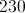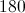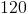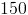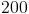Correct answer:Explanation:

If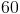minutes of Biology (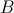) is twice as much time as that which is spent on Math (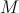), then Math must equal 30 minutes.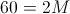, so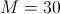.minutes is equivalent toof the total time ():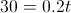Solve forby dividing both sides of the equation by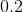.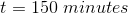### Example Question #1 : How To Find The Whole From The Part With Percentage

A bowl containsmarbles that are either red or green. The probability of selecting a red marble at random from the bowl isWhich of the following expressions represents the total number of red marbles in the bowl?

Possible Answers: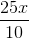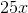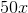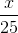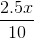Correct answer:Explanation:

It’s best to start with a fraction that is a part over 100. For this problem, we are looking for a fraction that shows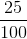.  If you go through the choices available,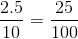. Therefore, if you plug in any value for “X” in this fraction, you will know the total number of marbles in the bowl. Here is an example:

If there are 4 marbles in the bowl, you’d expect 1 to be red. If we use this equation, we get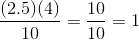### Example Question #31 : Percentage

Students at a country fair typically spend $40. The students have agreed to spend 40% less at this year's fair. Assuming all the students follow their new agreement, what is the most that could be spent by a student at this year's fair? Possible Answers:$10

$24$20

$30$16

Correct answer:

$24 Explanation: This is a percentage problem, so it is important to know that 40% of some value is equal to 0.4 of said value. So to find 40% of$40, we multiply 40 * 0.40, which equals 16.  This 16 represents 40% of the original value of $40. So if a student was to spend 40% less than they usually spend, we would subtract$16, which is 40% of the original value, from $40, giving us an answer of$24.

### Example Question #31 : Percentage

There are n pennies in a jar. Let p represent the decimal ratio of the pennies that are dated before 1983. Which of the following equations repesents the number of pennies that are dated 1983 or later?

Possible Answers:

n(1-p)

n(100-p)

np

n(1-p)/100

Correct answer:

n(1-p)

Explanation:

The proportion of pennies before 1983 would be np. Therefore, the pennies dated 1983 or later would be n(1-p).

### Example Question #1 : How To Find Decimal Equivalent To A Percentage

Express the following as a decimal: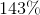Possible Answers:Correct answer:Explanation:

To convert a percentage to a decimal, you must add a decimal two points to the left from the right hand side. Thus,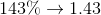### Example Question #2 : How To Find Decimal Equivalent To A Percentage

Which of the following is equivalent to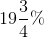?

Possible Answers:Correct answer:Explanation:

To find a decimal from a percentage, divide the percentage value by. In this case, convert the fraction to a decimal first: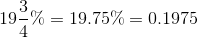### Example Question #3 : How To Find Decimal Equivalent To A Percentage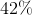share in a stock is added to a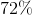share by speculators. What is the decimal equivalent of the combined shares?

Possible Answers:Correct answer:Explanation:

To convert from a percentage to a decimal, divide the decimal by. In this case, we can add the percentages first.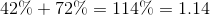### Example Question #1191 : Act Math

Sally sees a jacket on sale that's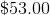. The store is having a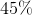storewide discount. What is the price of the jacket after the discount is applied?

Possible Answers: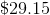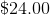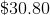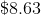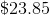Correct answer:Explanation:

The original price of the jacket is $53 and there's a discount applied that's close to being 50% off. Therefore we know the right answer should be a little greater than$26.50. This allows for the elimination of $24,$23.85 and $8.63 as options for viable answers. This problem requires transitioning percentages into decimals as currency. In this case, it's helpful to keep in mind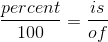. Using the information given, this skeleton would look like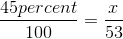. The intention of this is to see what 45% of$53.00 isThe is is what is being found, so it can be labeled as x. Using cross multiplication, x is calculated to be $23.85. X is what 45% of$53 is. Now the final calculation can be calculated through subtracting the x value from the jacket's original price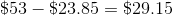.

### Example Question #2 : How To Find Percentage Equivalent To A Decimal

A high school senior takes a survey of the entire senior class for a project. She's interested in seeing the distribution of what her peers decide to pursue in college. She came up with the following data: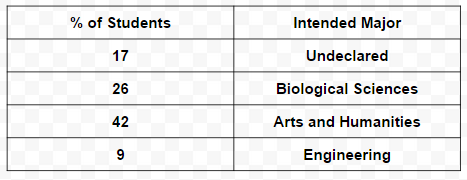The rest of the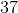students stated an interest in Linguistics. How many students in total were surveyed?

Possible Answers: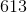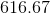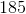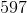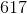Correct answer:Explanation:In this question, information about 5 groups of students is included but isn't expressed in the table nor is it expressed in terms of a percentage.

The sample the student took, despite how many students were interviewed, will have all the percents add up to 100% - as in the case with any study with percent breakdowns. The number of prospective Linguistic students is given (37), and it's know they're a part of the 100% the sample provides. To determine their percent, all the other intended majors percents must be subtracted from the total (100%).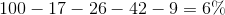Once this information has been uncovered, this problem becomes a matter of being able to calculate what 37 is 6% ofThis can be uncovered through the use of. In this question, the percent and "is" is known. The goal is to find what the "of" is. The of will provide the total number of students surveyed. Denoting the "of" as x, substituting all information in, and undergoing cross multiplication will provide the final answer.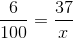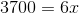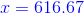Given that fractions of a person cannot be surveyed, the final answer must be rounded to the nearest whole number.

### Example Question #31 : Percentage

Which of the following is equivalent to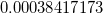?

Possible Answers: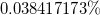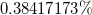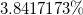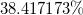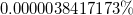Correct answer:Explanation:

To convert a decimal into a percent is fairly straightforward. Begin with your original decimal value:Then, move your decimal point to the right by two: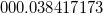Strip off any leading zeroes, add a percent sign, and you are good to go!### All ACT Math Resources